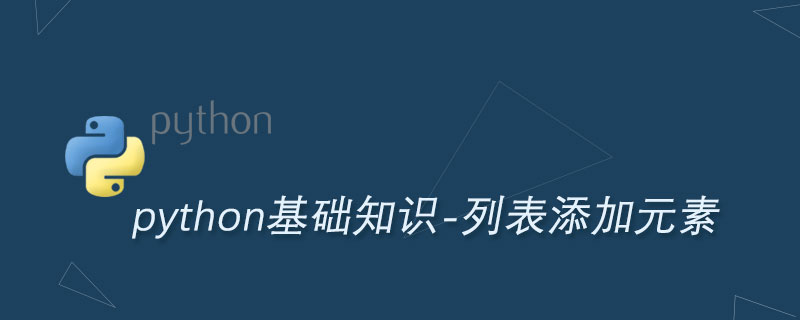Python list列表添加元素的3种方法Python list 列表增加元素可调用列表的 append() 方法，该方法会把传入的参数追加到列表的最后面。

append() 方法既可接收单个值，也可接收元组、列表等，但该方法只是把元组、列表当成单个元素，这样就会形成在列表中嵌套列表、嵌套元组的情形。例如如下代码：

a_list = ['crazyit', 20, -2]
# 追加元素
a_list.append('fkit')
print(a_list) # ['crazyit', 20, -2, 'fkit']
a_tuple = (3.4, 5.6)
# 追加元组，元组被当成一个元素
a_list.append(a_tuple)
print(a_list) # ['crazyit', 20, -2, 'fkit', (3.4, 5.6)]
# 追加列表，列表被当成一个元素
a_list.append(['a', 'b'])
print(a_list) # ['crazyit', 20, -2, 'fkit', (3.4, 5.6), ['a', 'b']]

['crazyit', 20, -2, 'fkit', (3.4, 5.6), ['a', 'b']]

b_list = ['a', 30]
# 追加元组中的所有元素
b_list.extend((-2, 3.1))
print(b_list) # ['a', 30, -2, 3.1]
# 追加列表中的所有元素
b_list.extend(['C', 'R', 'A'])
print(b_list) # ['a', 30, -2, 3.1, 'C', 'R', 'A']
# 追加区间中的所有元素
b_list.extend(range(97, 100))
print(b_list) # ['a', 30, -2, 3.1, 'C', 'R', 'A', 97, 98, 99]

c_list = list(range(1, 6))
print(c_list) # [1, 2, 3, 4, 5]
# 在索引3处插入字符串
c_list.insert(3, 'CRAZY' )
print(c_list) # [1, 2, 3, 'CRAZY', 4, 5]
# 在索引3处插入元组，元组被当成一个元素
c_list.insert(3, tuple('crazy'))
print(c_list) # [1, 2, 3, ('c', 'r', 'a', 'z', 'y'), 'CRAZY', 4, 5]
Python开发开发项目